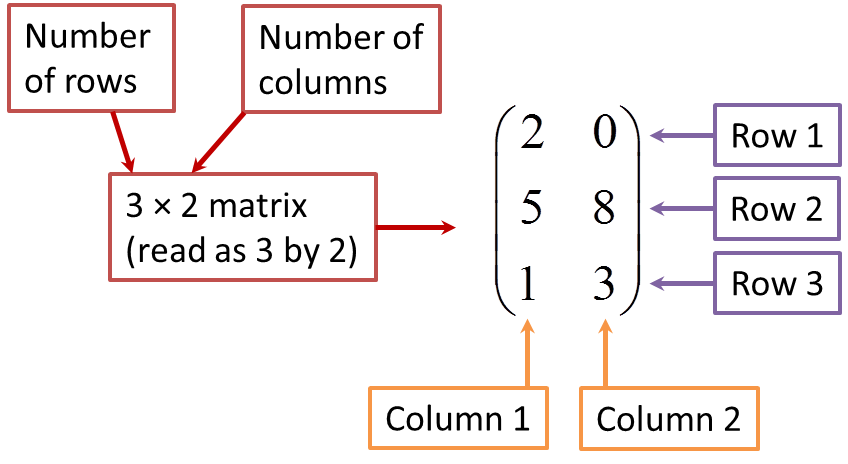# 4.1 Matrices

4.1 Matrices

A matrix is a rectangular array of numbers enclosed in large brackets.
$\text{For example}\left(\begin{array}{cc}2& 0\\ 3& 1\end{array}\right)\text{is a matrix}\text{.}$

(A)   Rows, Columns and Order of Matrices
1. A matrix which has m rows and columns is known as a matrix of order m × n.2. A row matrix is a matrix which has only one row.
Example:

3. A column matrix is a matrix which has only one column.
Example:

4. A square matrix is a matrix which has equal number of rows and columns.

Example: